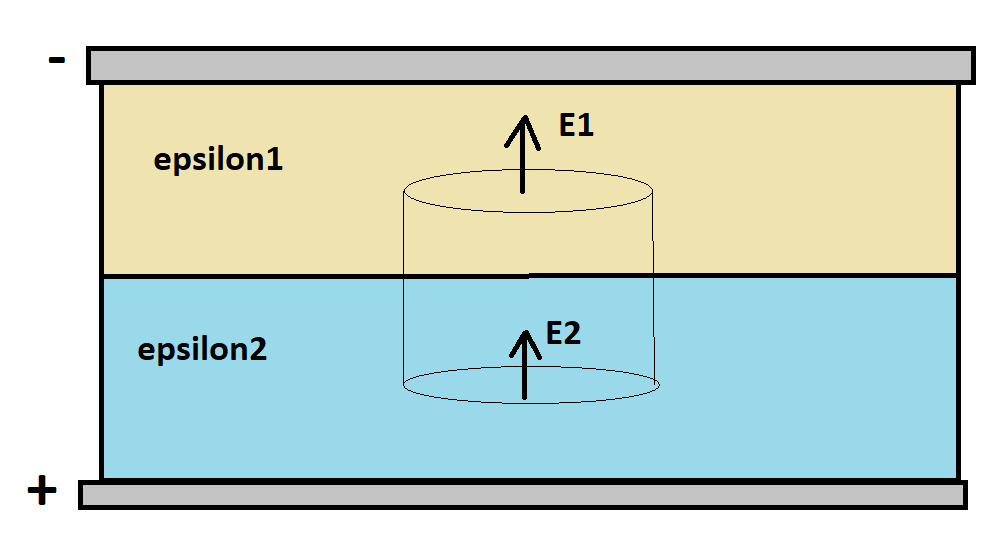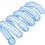# Gauss's Law / Dielectric BoundaryConsider a charged capacitor with two different dielectric materials, as shown. The electric fields point from the positive plate to the negative plate. Determine the relationship between the electric fields $E_1$ and $E_2$.

Draw a Gaussian surface around the dielectric boundary as shown, and apply Gauss's law to the closed surface.

$\int \int \epsilon \vec{E} \cdot \vec{dS} = Q$

In the above equation, $Q$ is the enclosed free charge at the dielectric interface. In the ordinary "text book" case, $Q = 0$. Integrating over the top and bottom surfaces results in (assuming the top and bottom surfaces have area $A$):

$\epsilon_1 E_1 A - \epsilon_2 E_2 A = 0 \\ \epsilon_1 E_1 = \epsilon_2 E_2$Note by Steven Chase
8 months, 1 week ago

This discussion board is a place to discuss our Daily Challenges and the math and science related to those challenges. Explanations are more than just a solution — they should explain the steps and thinking strategies that you used to obtain the solution. Comments should further the discussion of math and science.

When posting on Brilliant:

• Use the emojis to react to an explanation, whether you're congratulating a job well done , or just really confused .
• Ask specific questions about the challenge or the steps in somebody's explanation. Well-posed questions can add a lot to the discussion, but posting "I don't understand!" doesn't help anyone.
• Try to contribute something new to the discussion, whether it is an extension, generalization or other idea related to the challenge.
• Stay on topic — we're all here to learn more about math and science, not to hear about your favorite get-rich-quick scheme or current world events.

MarkdownAppears as
*italics* or _italics_ italics
**bold** or __bold__ bold
- bulleted- list
• bulleted
• list
1. numbered2. list
1. numbered
2. list
Note: you must add a full line of space before and after lists for them to show up correctly
paragraph 1paragraph 2

paragraph 1

paragraph 2

[example link](https://brilliant.org)example link
> This is a quote
This is a quote
    # I indented these lines
# 4 spaces, and now they show
# up as a code block.

print "hello world"
# I indented these lines
# 4 spaces, and now they show
# up as a code block.

print "hello world"
MathAppears as
Remember to wrap math in $$ ... $$ or $ ... $ to ensure proper formatting.
2 \times 3 $2 \times 3$
2^{34} $2^{34}$
a_{i-1} $a_{i-1}$
\frac{2}{3} $\frac{2}{3}$
\sqrt{2} $\sqrt{2}$
\sum_{i=1}^3 $\sum_{i=1}^3$
\sin \theta $\sin \theta$
\boxed{123} $\boxed{123}$

## Comments

Sort by:

Top Newest

@Talulah Riley I will be traveling most of the day today, so this might be the last thing I post

- 8 months, 1 week ago

Log in to reply

@Steven Chase where you are travelling? For your work or chilling your life??

- 8 months, 1 week ago

Log in to reply

@Steven Chase Thanks for the note.

- 8 months, 1 week ago

Log in to reply

×

Problem Loading...

Note Loading...

Set Loading...